# Calendar dates#### Everything You Need in One Place

Homework problems? Exam preparation? Trying to grasp a concept or just brushing up the basics? Our extensive help & practice library have got you covered.#### Learn and Practice With Ease

Our proven video lessons ease you through problems quickly, and you get tonnes of friendly practice on questions that trip students up on tests and finals.#### Instant and Unlimited Help

Our personalized learning platform enables you to instantly find the exact walkthrough to your specific type of question. Activate unlimited help now!

0/5
##### Intros
###### Lessons
1. Introduction to Calendar Dates:
2. What are calendar dates?
3. Written form of dates
4. Metric notation of dates
5. Explaining leap years (and the number of days in each month)
6. Other number formats/notations for dates
0/13
##### Examples
###### Lessons
1. Writing calendar dates in metric notation
Write the date in metric notation
1. June 29th, 1910
2. March 1st, 2004
3. October 16th, 2012
2. Writing metric notation dates in written (standard) form
Write the metric notation date in written form, using words and numbers
1. 1970 12 02
2. 2119 09 15
3. 2028 01 23
3. Writing calendar dates in different number notations
Write the calendar date shown in different notation formats:

1.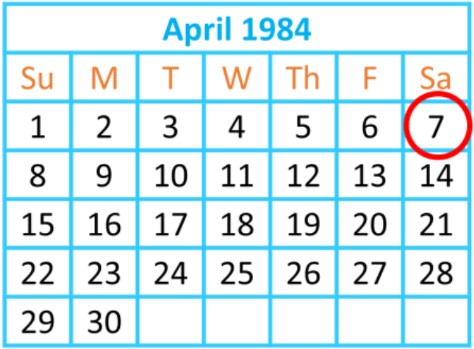1. $\overline{Y}$ $\overline{Y}$ $\;$ $\overline{M}$ $\overline{M}$ $\;$ $\overline{D}$ $\overline{D}$
2. $\overline{D}$ $\overline{D}$ $\;$ $\overline{M}$ $\overline{M}$ $\;$ $\overline{Y}$ $\overline{Y}$
3. $\overline{M}$ $\overline{M}$ $\;$ $\overline{D}$ $\overline{D}$ $\;$ $\overline{Y}$ $\overline{Y}$

2.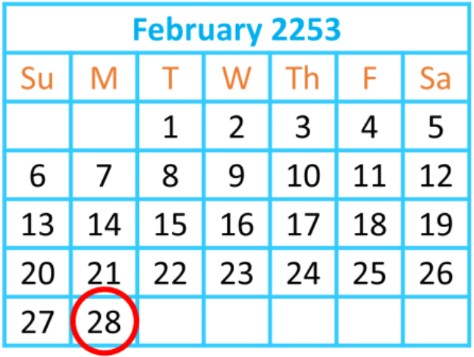1. $\overline{Y}$ $\overline{Y}$ $\;$ $\overline{M}$ $\overline{M}$ $\;$ $\overline{D}$ $\overline{D}$
2. $\overline{D}$ $\overline{D}$ $\;$ $\overline{M}$ $\overline{M}$ $\;$ $\overline{Y}$ $\overline{Y}$
3. $\overline{M}$ $\overline{M}$ $\;$ $\overline{D}$ $\overline{D}$ $\;$ $\overline{Y}$ $\overline{Y}$

3.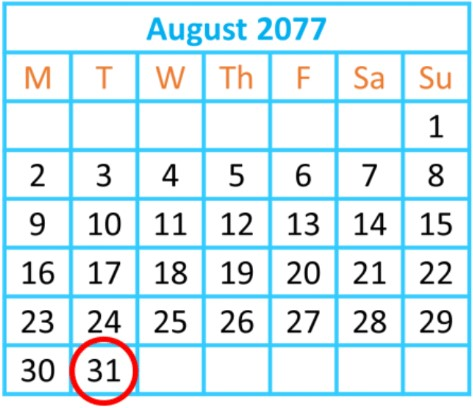1. $\overline{Y}$ $\overline{Y}$ $\;$ $\overline{M}$ $\overline{M}$ $\;$ $\overline{D}$ $\overline{D}$
2. $\overline{D}$ $\overline{D}$ $\;$ $\overline{M}$ $\overline{M}$ $\;$ $\overline{Y}$ $\overline{Y}$
3. $\overline{M}$ $\overline{M}$ $\;$ $\overline{D}$ $\overline{D}$ $\;$ $\overline{Y}$ $\overline{Y}$
4. Calendar Dates - Word Problem 1
Writing dates can be confusing!
Angie wrote her birth date on paper: 11 25 98
1. Can these numbers represent more than one date?
Write all possibilities for her birthday in written form.
2. Angie's sister Mina was born 3 years, one month, and 5 days later.
Write Mina's birthday in written form and in metric notation.
5. Calendar Dates - Word Problem 2
Writing dates can be confusing!
Toby wrote his birth date on paper: 01 07 13
1. Can these numbers represent more than one date?
Write all possibilities for his birthday in written form.
2. Toby wrote his birth date on paper: 01 07 13
If Toby told you that he was born during the summer of 2013, what is his birthday in metric notation?
###### Topic Notes

In this lesson, we will learn:

• How to write calendar dates in written form (words, ordinals, and numbers)
• How to write calendar dates in metric notation (numbers only, in the format: yyyy/mm/dd)
• Writing calendar dates using numbers in a variety of formats (mm/dd/yyyy OR mm/dd/yy OR dd/mm/yy)

Notes:

• Calendars and dates keep track of time! A date is a reference to a specific day in time, and we can tell how much time passes between two dates.
• Calendar dates use the units of time for: month, day, and year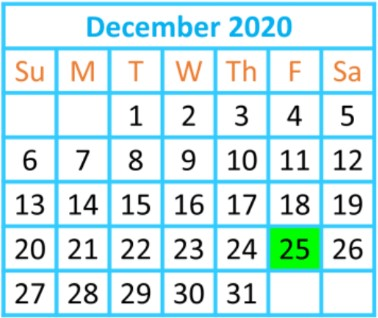• The written form of a calendar date is in the format: [month] [day]ordinal, [year]
• Ex. December 25th, 2020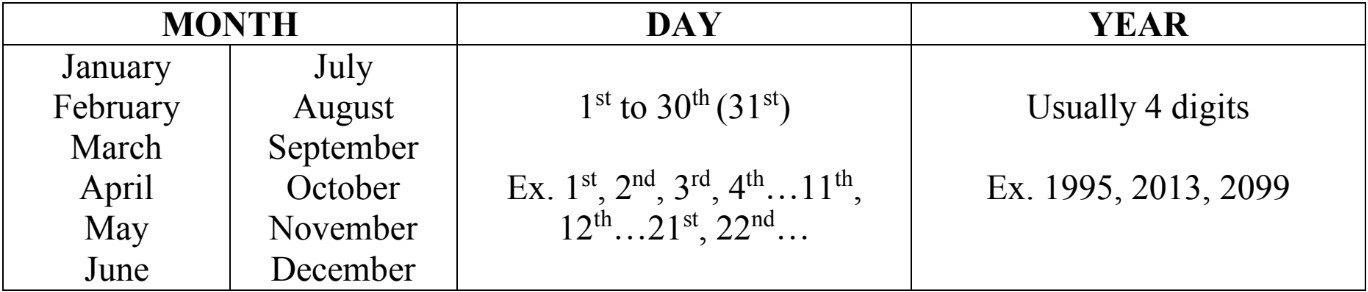• The metric notation of a calendar date is in the order: year / month / date
• i.e. yyyy / mm / dd (sometimes it is seen with dashes or spaces instead)
• this orders the time units from longest to shortest durations
• Ex. 2020 / 12 / 25 is December 25th, 2020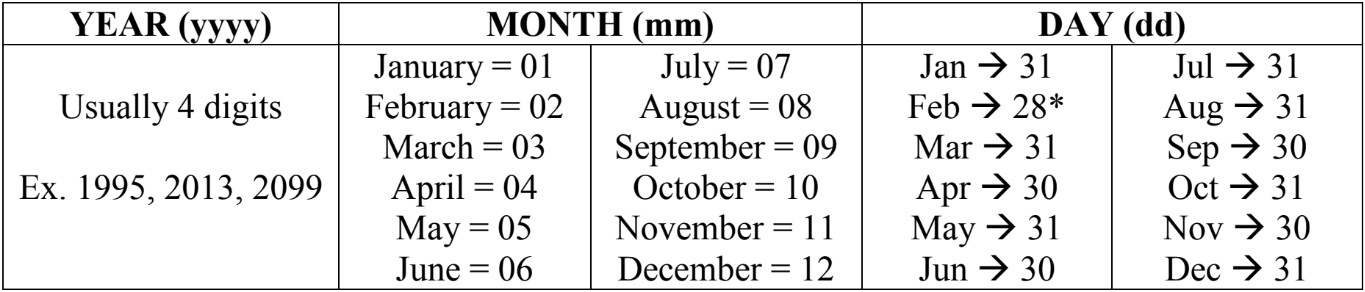• *A special exception is that February has 29 days (instead of 28 days) during a leap year (every 4 years).

• Dates in metric notation are used often when filling in paperwork!
• There are other formats of writing out the date using numbers:
• mm/dd/yyyy (different order)
• mm/dd/yy (only the last 2 digits of the year)
• dd/mm/yy (different order)